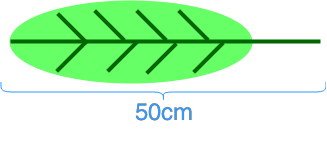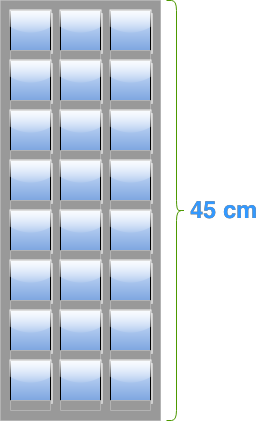# Scale diagrams#### Everything You Need in One Place

Homework problems? Exam preparation? Trying to grasp a concept or just brushing up the basics? Our extensive help & practice library have got you covered.#### Learn and Practice With Ease

Our proven video lessons ease you through problems quickly, and you get tonnes of friendly practice on questions that trip students up on tests and finals.#### Instant and Unlimited Help

Our personalized learning platform enables you to instantly find the exact walkthrough to your specific type of question. Activate unlimited help now!

0/1
##### Intros
###### Lessons
1. What is a scale factor?
0/4
##### Examples
###### Lessons
1. What is the actual length of each object?
1. The scale for the image of a leave is 1: 0.2.2. The scale for the image of a building is 1: 4062. Tony is 6 cm tall in a photo. Yet, his actual height is 1.86 m. What is the scale factor?
1. A spaceship is 56 m in height. You have built a model of the spaceship with a scale factor of 1:60 for your cousin overseas. However, the longest shipping box you can find is only 0.8 m. Will the spaceship model fit into the shipping box?
###### Free to Join!
StudyPug is a learning help platform covering math and science from grade 4 all the way to second year university. Our video tutorials, unlimited practice problems, and step-by-step explanations provide you or your child with all the help you need to master concepts. On top of that, it's fun - with achievements, customizable avatars, and awards to keep you motivated.
• #### Easily See Your ProgressWe track the progress you've made on a topic so you know what you've done. From the course view you can easily see what topics have what and the progress you've made on them. Fill the rings to completely master that section or mouse over the icon to see more details.
• #### Make Use of Our Learning Aids###### Practice Accuracy

Get quick access to the topic you're currently learning.

See how well your practice sessions are going over time.

Stay on track with our daily recommendations.

• #### Earn Achievements as You LearnMake the most of your time as you use StudyPug to help you achieve your goals. Earn fun little badges the more you watch, practice, and use our service.
• #### Create and Customize Your AvatarPlay with our fun little avatar builder to create and customize your own avatar on StudyPug. Choose your face, eye colour, hair colour and style, and background. Unlock more options the more you use StudyPug.
###### Topic Notes
What is the actual length of a street on the map? All we need is a ruler and the map's scale factor! By using the scale factors, we can easily find the actual size of an object. See how to do that in this lesson.

## What is a scale factor

Diagrams that you see may not always be drawn to its intended exact size. Instead, it may be scaled up to help you see the diagram better, or it may be scaled down so that it can fit on the medium that you're seeing the diagram on. Whichever it may be, scale diagrams may be drawn to a certain scale factor.

Scale factors are the ratio of two corresponding measurements on two similar figures. It is used in scaling and isn't constrained to 2D shapes. You can also use a scale factor with 3D shapes. If you have the scale factor, you can convert a measurement into another similar figure's measurements. An example of this in use is when you're changing a diagram on a paper's measurements to the real-life item.

## How to find scale factor

Let's say you've got two figures: figure A and figure B. They are both similar, and figure B is an enlargement of figure A. How do you go about finding the scale factor between the two?

The scale factor, as a reminder, is a ratio, so what we're looking for is a ratio that can relate figure A and B. You can calculate the scale factor when you've got an enlargement by putting a side of the bigger figure divided by the smaller figure. That is:

big/small

In this case, it would mean that the scale factor of the enlargement = $\frac{B side}{A side}$.

Alternatively, if you were looking at the scale factor of a reduction you'd put the smaller figure's side over the bigger figure's side. That is:

small/big

## Example problems

See if you can tackle the below scale factor examples that deal with scale drawings and put into use what you know about the scale factor definition!

Question 1:

What is the actual length of this object?

The scale for the image of a leaf is 1 : 0.2.The scale for the image of a leaf

Solution:

$ratio = \frac{image}{actual} = \frac{1}{0.2} = 50cm$

Find the unknown:

$\frac{1 \times 50}{0.2 \times 50} = \frac{50cm}{10cm}$

10cm is the actual length of the leaf.

Question 2:

What is the actual length of this object?

The scale for the image of a building is 1: 406The scale for the image of a building

Solution:

$ratio = \frac{image}{actual} = \frac{1}{406} = 45cm$

Find the unknown:

$\frac{1 \times 45}{406 \times 45} = \frac{45cm}{18270cm}$

18270cm is the actual height of the building.

Question 3:

Tony is 6cm tall in a photo. Yet, his actual height is 1.86 m. What is the scale factor?

Solution:

$scale factor = \frac{image}{actual}$

First, we need to convert unit:

$1.86m \times \frac{100cm}{1m} = 186cm$

So, put numbers in $scale factor = \frac{image}{actual}$

$scale factor = \frac{6cm}{186cm}$

Simplify it and we get the final answer

$\frac{1}{31}$